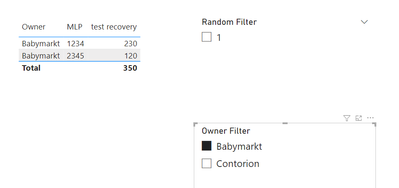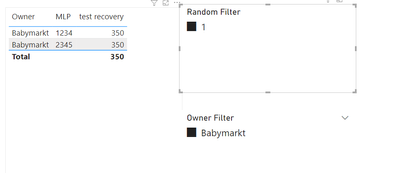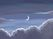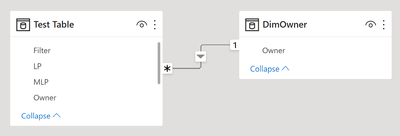cancel
Showing results for
Did you mean:

Fabric is Generally Available. Browse Fabric Presentations. Work towards your Fabric certification with the Cloud Skills Challenge.

## SUMX producing wrong result when filtered in both row context and filter context

Hi all!

Sorry for the title, I just have no good way of explaining this issue. Example file: https://drive.google.com/file/d/1lc-GdEEYAMYjqrm1YfVm9wuWE9Ls_gRq/view?usp=sharing

I have a table like this:LP is the lowest granularity. MLP is the "master" LP. So 2 LP's can be part of 1 MLP.
Recovery is a value that is stored on each row of this table, but when summing it, you are only supposed to take it once for each value of MLP, not for each value of LP.
Finally, I create a random filter, something that splits the rows. I dont really care about this filter, but I need it to trigger my issue.

I created this measure:

test recovery =
SUMX(
VALUES('Test Table'[MLP]),
CALCULATE(MAX('Test Table'[Recovery]))
)

The idea being using MLP as the iterator while calulating the max of recovery for each MLP, and then summing those amounts.

I then create the following table, with these filters:The result is exactly as expected. The total works, the values work, great.

Then I filter on the owner babymarkt:Still perfect results.

Now I'm going to filter on my random filter that should, as far as I understand it, have no effect, because all rows of babymarkt have filter value 1:The totals rows are still correct, but the individual MLP rows are no longer, and now show the totals too.
When I remove the filter on Owner, the results are as expected again.

Something seems to be happening with the owner filter. When I filter for owner only through the row context, it works fine, but when I filter through both the row and filter context, it messes up somehow once I add any other filter.

I am not interested in solving this issue (I have received some good suggestions already), but I am interested in understanding why SUMX messes up in this situation, and if, possibly, I have found a bug in DAX that I can use to impress Marco & Alberto with 😉

Thanks!

Status: Investigating

SUMX is an iteration function in Power BI that works on a row-by-row calculation per the given expression or equation. This function considers each row at a time and applies the calculation. It will not concentrate on the entire column, unlike the SUM function. But, it works like a cell be cell formula in Excel. SUM is an aggregate function, and SUMX is an expression function. One can perform Power BI data manipulation by using DAX functions, and SUMX is one such function in Power BI.

The SUMX function takes as its first argument a table, or an expression that returns a table. The second argument is a column that contains the numbers you want to sum, or an expression that evaluates to a column.

• Only the numbers in the column are counted. Blanks, logical values, and text are ignored.

• For more complex examples of SUMX in formulas, see ALL and CALCULATETABLE.

• This function is not supported for use in DirectQuery mode when used in calculated columns or row-level security (RLS) rules.Community Support
Status changed to: Investigating

SUMX is an iteration function in Power BI that works on a row-by-row calculation per the given expression or equation. This function considers each row at a time and applies the calculation. It will not concentrate on the entire column, unlike the SUM function. But, it works like a cell be cell formula in Excel. SUM is an aggregate function, and SUMX is an expression function. One can perform Power BI data manipulation by using DAX functions, and SUMX is one such function in Power BI.

The SUMX function takes as its first argument a table, or an expression that returns a table. The second argument is a column that contains the numbers you want to sum, or an expression that evaluates to a column.

• Only the numbers in the column are counted. Blanks, logical values, and text are ignored.

• For more complex examples of SUMX in formulas, see ALL and CALCULATETABLE.

• This function is not supported for use in DirectQuery mode when used in calculated columns or row-level security (RLS) rules.Hi there, thanks for the response.

I did already know how it's supposed to work. In my case, SUMX should do exactly what I need (SUM aggregate the recovery on a MAX basis, iterating over the MLP column). Unfortunately, it stops working when applying the filters as described in my example. Did you test the example file?MVP

So far, you impressed me 🙂

I don't have a decent answer yet, I am investigating on this.

I will keep you posted.

Have fun with DAX!

Alberto Ferrari
http://www.sqlbi.comResident Rockstar

I am interested to know what suggestions you received that fixed the problem.

Looking at the DAX, I was wondering why you needed to include the CALCULATE within the SUMX expression.  I tried to remove it, but found that it broke the Sub-Total.The Sub-Total without CALCULATE does not work because the VALUES function creates a table with 2 rows - both with the max value of 230 - hence the SUM = 460.

When you add the CALCULATE it appears to change the filter context, the VALUES contains 2 rows with max value of 120 and 230, so the SUM is correct.

Unfortunately, including CALCULATE breaks the filter context when start to include addition fitler without include the additional filter in the visual ROW Context.  Consider the following examples.VersusIt appears in the execution plan the the MAX Function is considering the Owner and Filter columns only without applying MLP filter, so it sums 120 and 230 as VALUES includes both MLP.

``````// DAX Query
DEFINE
VAR __DS0FilterTable =
TREATAS({"1"}, 'Test Table'[Filter])

VAR __DS0FilterTable2 =
TREATAS({"Babymarkt"}, 'Test Table'[Owner])

VAR __DS0Core =
SUMMARIZECOLUMNS(
__DS0FilterTable,
__DS0FilterTable2,
"test_recovery", 'Test Table'[test recovery]
)

VAR __DS0PrimaryWindowed =
TOPN(502, __DS0Core, [IsGrandTotalRowTotal], 0, 'Test Table'[Owner], 1, 'Test Table'[MLP], 1)

EVALUATE
__DS0PrimaryWindowed

ORDER BY
[IsGrandTotalRowTotal] DESC, 'Test Table'[Owner], 'Test Table'[MLP]``````

The following show how the CALCULATE is removing the MLP filter context for the Rows.

``````DEFINE
MEASURE 'Test Table'[test recovery] =
SUMX (
VALUES ( 'Test Table'[MLP] ),
CALCULATE ( MAX ( 'Test Table'[Recovery] ) )
)
MEASURE 'Test Table'[test count] =
SUMX (
VALUES ( 'Test Table'[MLP] ),
CALCULATE ( COUNTA ( 'Test Table'[MLP] ) )
)
MEASURE 'Test Table'[test no calculate] =
SUMX (
VALUES ( 'Test Table'[MLP] ),
MAX ( 'Test Table'[Recovery] )
)
MEASURE 'Test Table'[test count no calculate] =
SUMX (
VALUES ( 'Test Table'[MLP] ),
COUNTA ( 'Test Table'[MLP] )
)

EVALUATE
SUMMARIZECOLUMNS (
'Test Table'[Owner],
'Test Table'[MLP],
TREATAS ( { "1" }, 'Test Table'[Filter] ),
TREATAS ( { "Babymarkt" }, 'Test Table'[Owner] ),
"test_recovery", 'Test Table'[test recovery],
"test_count", 'Test Table'[test count],
"test_no calculate", 'Test Table'[test no calculate],
"test_count_no calculate", 'Test Table'[test count no calculate]
)

EVALUATE
SUMMARIZECOLUMNS (
'Test Table'[Owner],
'Test Table'[MLP],
'Test Table'[Filter],
TREATAS ( { "1" }, 'Test Table'[Filter] ),
TREATAS ( { "Babymarkt" }, 'Test Table'[Owner] ),
"test_recovery", 'Test Table'[test recovery],
"test_count", 'Test Table'[test count],
"test_no calculate", 'Test Table'[test no calculate],
"test_count_no calculate", 'Test Table'[test count no calculate]
)``````Hi there!

Thanks a lot for your responses! Feels good to know that I'm not going crazy 🙂

So first off, I got this reponse from first posting it on the forums. This solution works in this case, but it's creating a whole new table under water, so it feels like taking out an ant with a ballistic missile 😛

Re: SUMX issue when having the same filter in the ... - Microsoft Power BI Community

The reason I used the CALCULATE is to trigger context transition. I need the row context in the calculation to only contain the MLP that the iterator is currently looking at. In order to do that, you have to use CALCULATE.

Your later investigation is correct. Adding the filter as a column removes the issue. The same happens when you remove the owner field from your table, or remove the owner slicer selection. Suddely it works as expected again. It really seems to have something to do with the owner field being part of the slicer and the visual itself. I think that's what's tripping it up.

You say that "The following show how the CALCULATE is removing the MLP filter context for the Rows.", but shouldn't the SUMMARIZE here solve that by aggregating toward the owner and MLP? I'm no DAX expert though, especially not when working with the "DAX behind the DAX", heheh.

When I look at your two pieces of code:

``````EVALUATE
SUMMARIZECOLUMNS (
'Test Table'[Owner],
'Test Table'[MLP],
TREATAS ( { "1" }, 'Test Table'[Filter] ),
TREATAS ( { "Babymarkt" }, 'Test Table'[Owner] ),
"test_recovery", 'Test Table'[test recovery],
"test_count", 'Test Table'[test count],
"test_no calculate", 'Test Table'[test no calculate],
"test_count_no calculate", 'Test Table'[test count no calculate]
)

EVALUATE
SUMMARIZECOLUMNS (
'Test Table'[Owner],
'Test Table'[MLP],
'Test Table'[Filter],
TREATAS ( { "1" }, 'Test Table'[Filter] ),
TREATAS ( { "Babymarkt" }, 'Test Table'[Owner] ),
"test_recovery", 'Test Table'[test recovery],
"test_count", 'Test Table'[test count],
"test_no calculate", 'Test Table'[test no calculate],
"test_count_no calculate", 'Test Table'[test count no calculate]
)``````

I cannot imagine what would make the first one not work and the second one work. The MLP field is treated exactly the same in both cases.Resident Rockstar

Thanks @jaap_olsthoorn - I think that using SUMMARIZE would be recommended over the use of VALUES and CALCULATE.  The suggestion to use Two measures was a little odd.  @amitchandak suggestion was much better.

This is what I would have used:

``````test recovery =
SUMX (
SUMMARIZE (
'Test Table',
'Test Table'[MLP],
"Max", MAX ( 'Test Table'[Recovery] )
),
[Max]
)``````Maybe this will be a good video for @AlbertoFerrari to highlight when to use VALUES versus SUMMARIZE.MVP

Sorry, Daryl, but NO.

SUMMARIZE is the source of most obscure numbers. I wrote about it several times, the last one was here: All the secrets of SUMMARIZE - SQLBI. The suggestion is the same since the beginning of DAX: do NOT use SUMMARIZE to compute measures, unless you love spending nights debugging your code.

I am still investigating on the topic, and it could take a long while. It seems to be a really weird scenario where auto-exists creates issues, as it might be a bug somewhere in the engine.

The original formula is just perfect: an iteration, context transition, an aggregation. There are multiple workarounds, even though they are what they are: workarounds. The formula, by itself, should already work.

For example, adding KEEPFILTERS around VALUES reduces the problems of auto-exists and makes the formula work:

``````test recovery =
SUMX(
KEEPFILTERS ( VALUES('Test Table'[MLP] ) ),
CALCULATE(MAX('Test Table'[Recovery]))
)``````

Another simple solution requires using a variable:

``````Using Addcolumns =
VAR A =
VALUES ( 'Test Table'[MLP] ),
"@Result", CALCULATE ( MAX ( 'Test Table'[Recovery] ) )
)
RETURN
SUMX ( A, [@Result] )``````

Nonetheless, despite working, these are workarounds. I still don't know exactly the reason for that behavior, therefore I cannot suggest one solution against the other.

Have fun with DAX!

Alberto Ferrari
http:Yea like I said, his solution works. I'm just super curious why the values or distinct version does not, because in most cases (where you dont have the owner in a filter AND in a visual) it works just fine! As far as I can tell, there is no good reason for SUMX to fail here...

Edit: Alberto gets it. It's not the solution I'm looking for, it's the explanation / bugfix 🙂Resident Rockstar

Thank you @AlbertoFerrari .  You have give some sound advice to consider in the future.  I didn't think that I was breaking your 1st principle, but I being to understand how the clustering would lead to problems.  It might works in this scenario, but not in others.  I also took the opportunity to read Macro's Auto-exist article.  I hope there is an answer to odd behaviour.Regular Visitor

I was very curious about this issue when I read it this morning and now I could have a look. Here's what I found:

I would like to begin with Alberto's own words:

"The golden rule of data modeling is always the same: always use star schemas."

I believe that these words should be written on the first report page in every new Power BI file created.

Then following these words, if you create an actual star schema instead of flat table, this issue is non-existent.

data model (DimOwner is created as distinct in Power Query from Test Table):and above data model produces this result when only adding new slicer from DimOwnerI understand that @jaap_olsthoorn  you're curious why is this happening, but for everyone finding this thread, in my opinion the best workaround is to just follow what Alberto and Marco are teaching us...

Always use star schemas

Wiliam Juricek

Completed Ideas